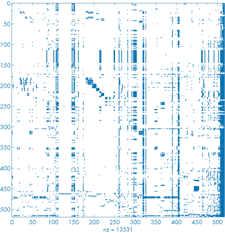# The World’s Largest Matrix Computation

By Cleve Moler, MathWorks

One of the reasons why Google is such an effective search engine is the PageRank™ algorithm, developed by Google's founders, Larry Page and Sergey Brin, when they were graduate students at Stanford University. PageRank is determined entirely by the link structure of the Web. It is recomputed about once a month and does not involve any of the actual content of Web pages or of any individual query. Then, for any particular query, Google finds the pages on the Web that match that query and lists those pages in the order of their PageRank.

Imagine surfing the Web, going from page to page by randomly choosing an outgoing link from one page to get to the next. This can lead to dead ends at pages with no outgoing links, or cycles around cliques of interconnected pages. So, a certain fraction of the time, simply choose a random page from anywhere on the Web. This theoretical random walk of the Web is a Markov chain or Markov process. The limiting probability that a dedicated random surfer visits any particular page is its PageRank. A page has high rank if it has links to and from other pages with high rank.

Let W be the set of Web pages that can reached by following a chain of hyperlinks starting from a page at Google and let n be the number of pages in W. The set W actually varies with time, but in May 2002, n was about 2.7 billion. Let G be the n-by-n connectivity matrix of W, that is, gi,j is 1 if there is a hyperlink from page i to page j and 0 otherwise. The matrix G is huge, but very sparse; its number of nonzeros is the total number of hyperlinks in the pages in W.

Let cj and ri be the column and row sums of G.

cj = Σ i gi,j,   ri= Σ j gi,j

The quantities ck and rk are the indegree and outdegree of the k-th page. Let p be the fraction of time that the random walk follows a link. Google usually takes p = 0.85. Then 1-p is the fraction of time that an arbitrary page is chosen. Let A be the n-by-n matrix whose elements are

ai,j = p gi,j / cj + δ, where δ = (1-p) / n.

The matrix A is not sparse, but it is a rank one modification of a sparse matrix. Most of the elements of A are equal to the small constant δ . When n = 2.7·109, δ = 5.5·10-11.

The matrix is the transition probability matrix of the Markov chain. Its elements are all strictly between zero and one and its column sums are all equal to one. An important result in matrix theory, the Perron-Frobenius Theorem, applies to such matrices. It tells us that the largest eigenvalue of A is equal to one and that the corresponding eigenvector, which satisfies the equation

x = Ax,

exists and is unique to within a scaling factor. When this scaling factor is chosen so that

Σ ixi = 1

then x is the state vector of the Markov chain. The elements of x are Google's PageRank.

If the matrix were small enough to fit in MATLAB, one way to compute the eigenvector x would be to start with a good approximate solution, such as the PageRanks from the previous month, and simply repeat the assignment statement

```x = Ax
```

until successive vectors agree to within specified tolerance. This is known as the power method and is about the only possible approach for very large n. I'm not sure how Google actually computes PageRank, but one step of the power method would require one pass over a database of Web pages, updating weighted reference counts generated by the hyperlinks between pages.Our PageRank example uses a tiny subset of the Web consisting of n = 517 pages on the MathWorks Web site, starting at the home page www.mathworks.com, and following 13,531 links. The `spy` plot of the connectivity matrix G shows many cliques of interconnected pages. The portion of the `spy` plot involving columns 508:517 contains 4013 links and so is fairly dense. These columns represent the bar of buttons on the top of the home page with labels like `products` and `support` and the small copyright button on the bottom of the page that are duplicated on many other pages. The buttons point to pages like www.mathworks.com/company/aboutus/policies_statements/trademarks.html. Rows 114:144 of the `spy` plot all have the same structure. These rows represent links from articles off the `pressroom` page to other pressroom material.

The PageRank calculation for this example produces a vector x with components that range between 0.0685 and 0.0003. Here are the top ten pages, together with their in and out degrees. We see that the pressroom has the highest rank, even though it has fewer references than some of the other pages. MATLAB Central, the new file exchange and newsgroup access page, is ranked fifth, and News & Notes is ranked seventh.

 PageRank in out URL `1` `0.068488` `57` `83` www.mathworks.com/company/pressroom `2` `0.027927` `452` `203` www.mathworks.com/help.html `3` `0.027923` `456` `46` www.mathworks.com/products `4` `0.010445` `1` `136` www.mathworks.com/products/ `5` `0.009961` `16` `34` www.mathworks.com/matlabcentral `6` `0.009916` `1` `123` www.mathworks.com/products/index.html?sec=alpha products/index.html?sec=alpha `7` `0.009206` `117` `36` www.mathworks.com/company/newsletters/news_notes/ `8` `0.008997` `2` `118` www.mathworks.com/products/ `9` `0.008343` `86` `42` www.mathworks.com/newsletters/company/digest `10` `0.008083` `423` `64` www.mathworks.com/supportPageRank of the small Web sample. The top three pages are the pressroom, the site index and the products button. Click on image to see enlarged view.Spy plot of the link structure of a small sample of the Web starting at www.mathworks.com. The dense columns on the right represent buttons that are common to many pages. Click on image to see enlarged view.

Published 2002